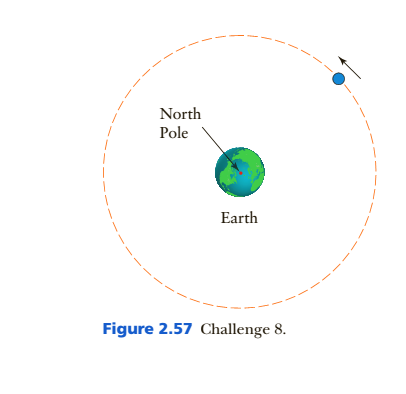Chapter 2, Problem 8C

Chapter
Section
Textbook Problem

A 200-k.g communications satellite is placed into a circular orbit around Earth with a radius of 4.23 107 m (26,300 miles) (Figure 2.57),(a) Find the gravitational force on the sateline. (There is some useful information in Section 2.7.) (b) use the equation for equation for to centripetal force the speed of the Stateline. (c) Show that the period of the Stateline −the time it takes to complete one orbit −is 1 day. (the distance it travels during one orbit is 2 ,6.8 times the radius.) This is a gtosynrhnnoiss orbit: the satellite stays above a fixed point on Earths equator.

To determine

(a)

The gravitational force on a satellite of mass 200-kg, orbiting the earth in a circular orbit of radius 4.23×107m.

Explanation

Given:

Mass of the satellite

m=200-kg

Radius of the satellite’s orbit

r=4.23×107m

Mass of the earth (from standard tables)

M=5.972×1024kg

Universal gravitational constant

G=6.67×1011N-m2/kg2.

Formula used:

The gravitational force between the satellite and the Earth is given by,

F=GMmr2.

Calculation:

Substitute the values of the variables in the formula and calculate the gravitational force.

F=GMmr2=(6

To determine

(b)

The speed of the satellite using the expression for the centripetal force.

To determine

(c)

The period of the satellite and show that it has a value equal to 1 day.

Still sussing out bartleby?

Check out a sample textbook solution.

See a sample solution

The Solution to Your Study Problems

Bartleby provides explanations to thousands of textbook problems written by our experts, many with advanced degrees!

Get Started

What is the most important chemical property of carboxylic acids?

Chemistry for Today: General, Organic, and Biochemistry

What is population genetics?

Human Heredity: Principles and Issues (MindTap Course List)

Crossing over mixes up ______. a. chromosomes b. alleles c. zygotes d. gametes

Biology: The Unity and Diversity of Life (MindTap Course List)